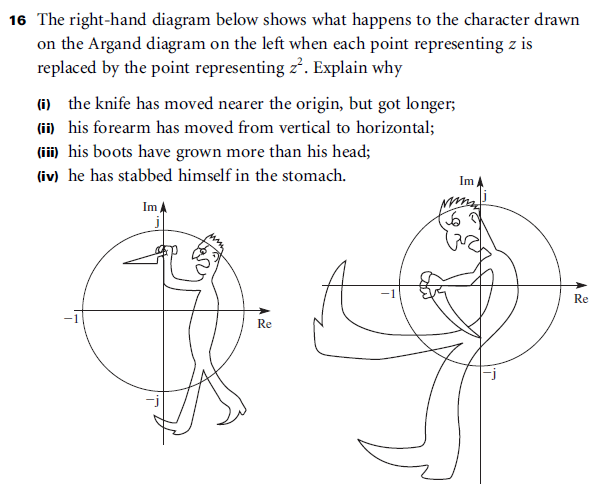Stabbing Man

Author:
Ben Sparks
Inspired by the MEI FP2 textbook - exercise 3C, question 16 (page 39) If the original 'figure' is a set of points in the complex plane, and they are transformed by the transformation z -> z², what does the result look like and why?

When/how to use

I use this file in the classroom when discussing the effect of multiplying complex numbers (i.e. you add the arguments and multiply the moduli). In particular, when you square a complex number you double the argument and square the modulus. This means things seem to rotate around the axis, and points outside the unit circle diverge while points inside converge (towards zero). It adds a humorous slant on the discussion, and provokes more questions (what if there were points on the left of the diagram originally, and what happens with other transformations, or other powers of z). For maximum effect: hide the original figure, and set the value of n -> 1 before you show anyone the diagram. Ask for conjectures on what happens to the image, and then press play. Original question here: